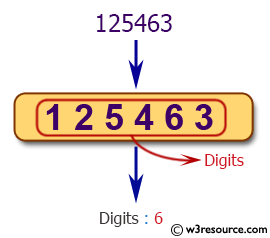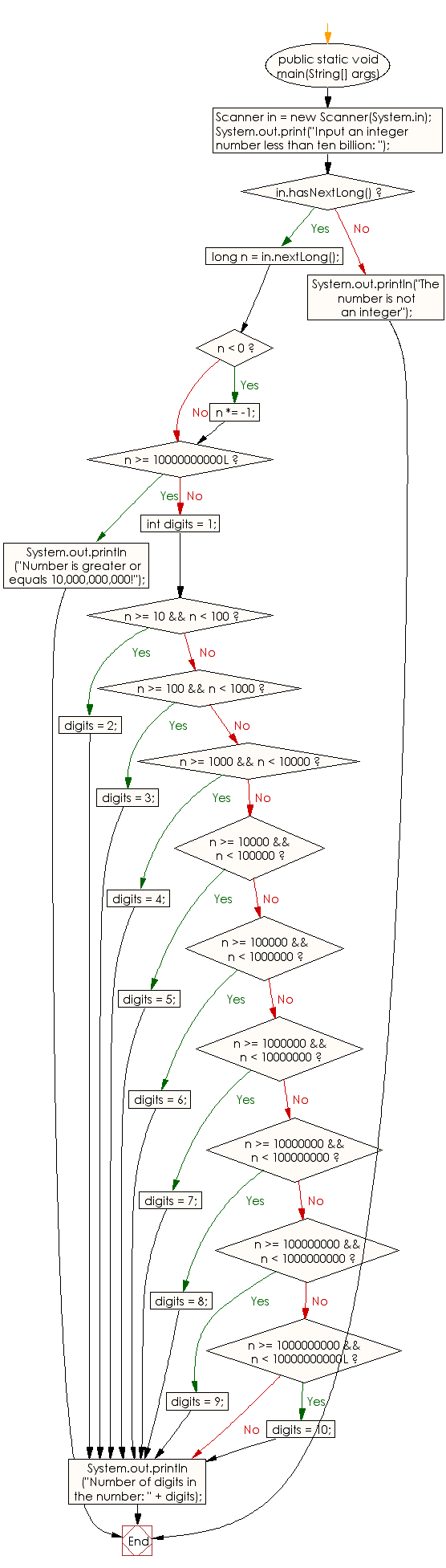﻿ Java exercises: Reads an positive integer and count the number of digits - w3resource# Java Conditional Statement Exercises: Reads an positive integer and count the number of digits

## Java Conditional Statement: Exercise-29 with Solution

Write a Java program that reads an positive integer and count the number of digits the number (less than ten billion) has.

Test Data
Input an integer number less than ten billion: 125463

Pictorial Presentation:Sample Solution:

Java Code:

``````import java.util.Scanner;
public class Exercise29 {

public static void main(String[] args)
{
Scanner in = new Scanner(System.in);
System.out.print("Input an integer number less than ten billion: ");

if (in.hasNextLong())
{

long n = in.nextLong();

if (n < 0)
{
n *= -1;
}
if (n >= 10000000000L)
{
System.out.println("Number is greater or equals 10,000,000,000!");
}
else
{
int digits = 1;
if (n >= 10 && n < 100)
{
digits = 2;
}
else if (n >= 100 && n < 1000)
{
digits = 3;
}
else if (n >= 1000 && n < 10000)
{
digits = 4;
}
else if (n >= 10000 && n < 100000)
{
digits = 5;
}
else if (n >= 100000 && n < 1000000)
{
digits = 6;
}
else if (n >= 1000000 && n < 10000000)
{
digits = 7;
}
else if (n >= 10000000 && n < 100000000)
{
digits = 8;
}
else if (n >= 100000000 && n < 1000000000)
{
digits = 9;
}
else if (n >= 1000000000 && n < 10000000000L)
{
digits = 10;
}
System.out.println("Number of digits in the number: " + digits);
}
}
else
{
System.out.println("The number is not an integer");
}
}
}
```
```

Sample Output:

```Input an integer number less than ten billion: 125463
Number of digits in the number: 6
```

Flowchart:Java Code Editor:

What is the difficulty level of this exercise?

﻿

## Java: Tips of the Day

How to split a String by space?

You can use the whitespace regex:

```str = "Hello I'm your String";
String[] splited = str.split("\\s+");
```

This will cause any number of consecutive spaces to split your string into tokens.

Ref: https://bit.ly/3xk42If